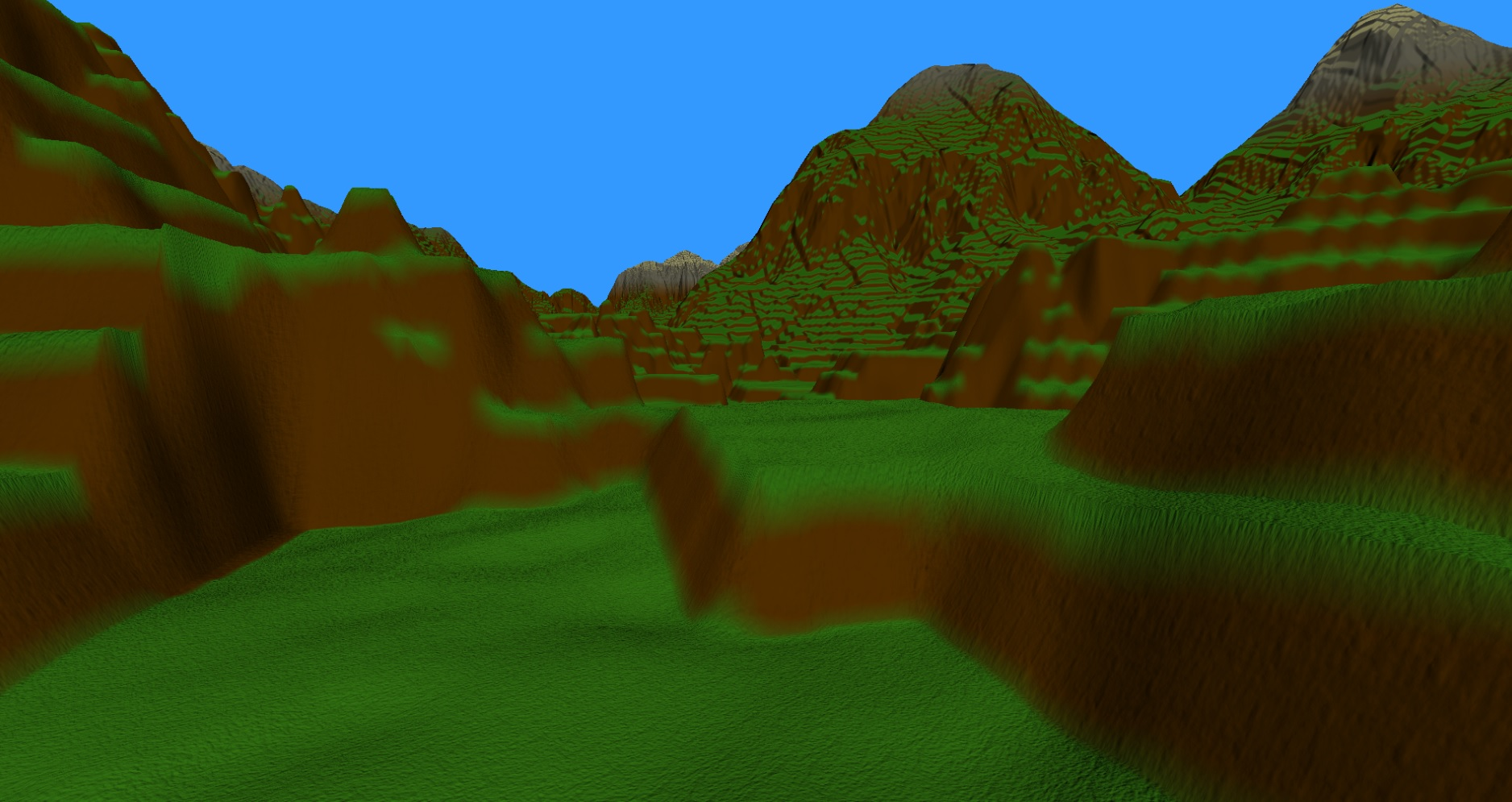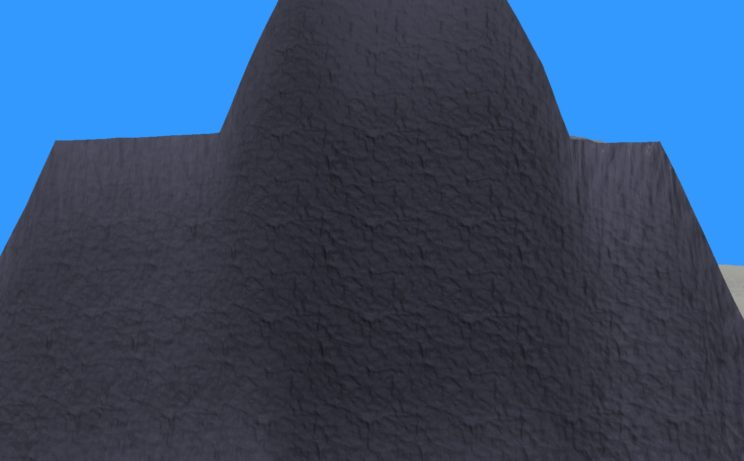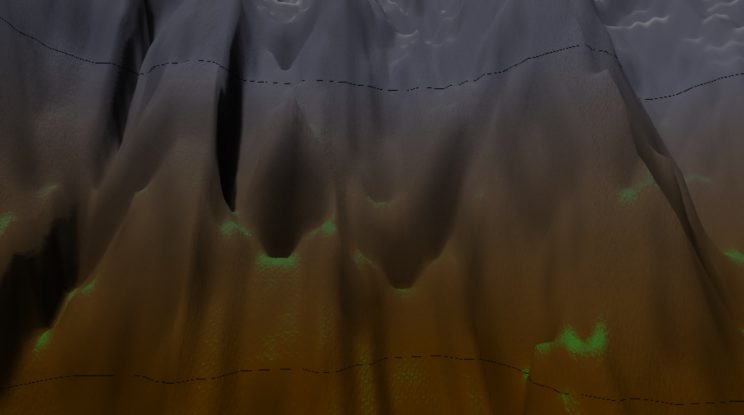# Rendering Terrain Part 24 – Height and Slope Based Normal Mapping

I finally figured out blending normal maps, and I’m reasonably happy with the results.

First off, I wanted to quickly revisit triplanar mapping. Originally, as discussed in Part 21, we had lessened texture stretching due to vertical surfaces quite a bit, but it wasn’t completely gone. Because we are blending three different samples together, the more off angle samples actually add some stretching into the final sample. This post addresses that by adding in a tightening factor. Our triplanar_sample() function now looks like this:

```float3 triplanar_sample(Texture2D tex, SamplerState sam, float3 texcoord, float3 N) {
float tighten = 0.4679f;
float3 blending = saturate(abs(N) - tighten);
// force weights to sum to 1.0
float b = blending.x + blending.y + blending.z;
blending /= float3(b, b, b);

float3 x = tex.Sample(sam, texcoord.yz).xyz;
float3 y = tex.Sample(sam, texcoord.xz).xyz;
float3 z = tex.Sample(sam, texcoord.xy).xyz;

return x * blending.x + y * blending.y + z * blending.z;
}```

And here is the result:Note: The texture is sampled at a different rate in this image than those in Part 21.

That looks quite a bit better in my opinion.

With that slight change, we can move forward with how I handle height and slope based normal mapping.
My first move was to decide to try and minimize my sampling. When the slope is less than a minimum threshold of steepness, we won’t bother taking multiple samples. So flat terrain requires only one texture sample as opposed to three. This, however, leads to the need to blend between that single sample value and a triplanar sample value for the steep areas. That blend region requires four samples. And anything steeper than the blend region requires three samples.

```float3 triplanar_slopebased_sample(float slope, float3 N, float3 V, float3 uvw, Texture2D texXY, Texture2D texZ, SamplerState sam) {
if (slope < 0.25f) {
return texZ.Sample(sam, uvw.xy).xyz;
}

float tighten = 0.4679f;
float3 blending = saturate(abs(N) - tighten);
// force weights to sum to 1.0
float b = blending.x + blending.y + blending.z;
blending /= float3(b, b, b);

float3 x = texXY.Sample(sam, uvw.yz).xyz;
float3 y = texXY.Sample(sam, uvw.xz).xyz;
float3 z = texXY.Sample(sam, uvw.xy).xyz;

if (slope < 0.5f) {
float3 z2 = texZ.Sample(sam, uvw.xy).xyz;

float blend = (slope - 0.25f) * (1.0f / (0.5f - 0.25f));

return lerp(z2, x * blending.x + y * blending.y + z * blending.z, blend);
}

return x * blending.x + y * blending.y + z * blending.z;
}```

I think overall this should be a reasonable way to do things, but it really requires further testing with different terrains.
One other possible way to do this was to simply perform normal triplanar sampling, but use texZ for the z axis. We’d be back to a static three samples no matter the slope and the function would be a lot simpler.

```float3 triplanar_sample(Texture2D texXY, Texture2D texZ, SamplerState sam, float3 texcoord, float3 N) {
float tighten = 0.4679f;
float3 blending = saturate(abs(N) - tighten);
// force weights to sum to 1.0
float b = blending.x + blending.y + blending.z;
blending /= float3(b, b, b);

float3 x = texXY.Sample(sam, texcoord.yz).xyz;
float3 y = texXY.Sample(sam, texcoord.xz).xyz;
float3 z = texZ.Sample(sam, texcoord.xy).xyz;

return x * blending.x + y * blending.y + z * blending.z;
}```

This method didn’t return as nice results:

I didn’t really notice any difference in performance between the two, although my testing was far from thorough.

The above code is just for sampling, so could easily be used with diffuse textures as well. To actually modify the final normal by this sample, we have a slightly modified perturb_normal function:

```float3 perturb_normal_slopebased_triplanar(float slope, float3 N, float3 V, float3 uvw, Texture2D texXY, Texture2D texZ, SamplerState sam) {
float3 map = triplanar_slopebased_sample(slope, N, V, uvw, texXY, texZ, sam);
map = 2.0f * map - 1.0f;

float3x3 TBN = cotangent_frame(N, -V, uvw);
return normalize(mul(map, TBN));
}```

The next stage is to apply this slope based mapping in height based bands that line up with the bands used for colour.

```float3 height_and_slope_based_normal(float height, float slope, float3 N, float3 V, float3 uvw) {
float bounds = scale * 0.02f;
float transition = scale * 0.6f;
float greenBlendEnd = transition + bounds;
float greenBlendStart = transition - bounds;
float snowBlendEnd = greenBlendEnd + 2 * bounds;

float3 N1 = perturb_normal_slopebased_triplanar(slope, N, V, uvw, detailmap2, detailmap1, displacementsampler);

if (height < greenBlendStart) {
// get grass/dirt values
return N1;
}

float3 N2 = perturb_normal_triplanar(N, V, uvw, detailmap3, displacementsampler);

if (height < greenBlendEnd) {
// get both grass/dirt values and rock values and blend
float blend = (height - greenBlendStart) * (1.0f / (greenBlendEnd - greenBlendStart));
return lerp(N1, N2, blend);
}

float3 N3 = perturb_normal_slopebased_triplanar(slope, N, V, uvw, detailmap3, detailmap4, displacementsampler);

if (height < snowBlendEnd) {
// get rock values and rock/snow values and blend
float blend = (height - greenBlendEnd) * (1.0f / (snowBlendEnd - greenBlendEnd));
return lerp(N2, N3, blend);
}

// get rock/snow values
return N3;
}```

You’ll notice that N2 uses the regular triplanar normal mapping instead of the new slope based method. That’s because I wanted a band of rock after the grass ends and before snow starts.
Something else that you’ll likely notice is that we perform the samples outside of the if statements. This means that as we move up in height, we accumulate samples. If we move the sampling into the if statements, we’d have some duplicate code because we need N1 within two different cases, but we don’t need the N1 sample at all in the last two cases, so why have it slowing us down?
Because of this:Notice the odd black lines across the top and bottom of the image? Those are where the separations are between the height bands. I can’t explain why, but simply moving the sampling into the if statements where if should cause less of a performance hit causes those lines. If not, my function would look like so:

```float3 height_and_slope_based_normal(float height, float slope, float3 N, float3 V, float3 uvw) {
float bounds = scale * 0.02f;
float transition = scale * 0.6f;
float greenBlendEnd = transition + bounds;
float greenBlendStart = transition - bounds;
float snowBlendEnd = greenBlendEnd + 2 * bounds;

if (height < greenBlendStart) {
// get grass/dirt values
float3 N1 = perturb_normal_slopebased_triplanar(slope, N, V, uvw, detailmap2, detailmap1, displacementsampler);
return N1;
}

if (height < greenBlendEnd) {
float3 N1 = perturb_normal_slopebased_triplanar(slope, N, V, uvw, detailmap2, detailmap1, displacementsampler);
float3 N2 = perturb_normal_triplanar(N, V, uvw, detailmap3, displacementsampler);
// get both grass/dirt values and rock values and blend
float blend = (height - greenBlendStart) * (1.0f / (greenBlendEnd - greenBlendStart));
return lerp(N1, N2, blend);
}

float3 N3 = perturb_normal_slopebased_triplanar(slope, N, V, uvw, detailmap3, detailmap4, displacementsampler);

if (height < snowBlendEnd) {
float3 N2 = perturb_normal_triplanar(N, V, uvw, detailmap3, displacementsampler);
// get rock values and rock/snow values and blend
float blend = (height - greenBlendEnd) * (1.0f / (snowBlendEnd - greenBlendEnd));
return lerp(N2, N3, blend);
}

// get rock/snow values
return N3;
}```

I wish I could figure out why those lines occur and get rid of them, because this version probably saves about 5ms per frame or more, depending on where we are looking. At this point, our frame rate isn’t terrible in the lower areas, but if we are looking at a larger portion where we are blending between regions and have as many as eleven samples per pixel, we can get up to 26ms, which drops us slightly below 30fps. Not good.

In order to help a bit with this cost, I decided to try something I had read often gets used in games: blending the normal mapping in based on distance. Basically, we don’t do as much, if any, normal mapping at a distance. I found I can blend the normals in as pixels get closer.

```float3 dist_based_normal(float height, float slope, float3 N, float3 V, float3 uvw) {
float dist = length(V);

float3 N1 = perturb_normal(N, V, uvw / 32, displacementmap, displacementsampler);

if (dist > 150) return N;

if (dist > 100) {
float blend = (dist - 100.0f) / 50.0f;

return lerp(N1, N, blend);
}

float3 N2 = height_and_slope_based_normal(height, slope, N1, V, uvw);

if (dist > 50) return N1;

if (dist > 25) {
float blend = (dist - 25.0f) / 25.0f;

return lerp(N2, N1, blend);
}

return N2;
}```

Again, I found that if I perform the sampling in the if blocks I get the same lines at the edges of the blend areas. So again I find myself making a potentially less efficient function in order to fix a problem that I don’t really understand. For instance, why does N1 need to be defined before the first if statement? If I even move that to just after the first if block, I’ll get a line.
Still, using this function reduces shimmering in the distance caused by pixels representing larger regions and their normals changing as you move. It also helped a bit with frame rate. Over much of the terrain, I can now get a frame rate of 10ms or less. Unfortunately, the worst case performance is not particularly improved by this. If you’ve got a large blend region in front of you, performance will be bad. This may simply indicate that my blend region is too large and should be reduced to as small a region as possible. I’ll have to mess with that later. We rarely, if ever, dip below 30fps, so I guess that’s something.

Which brings me to the end of this post. Next post, I hope to mess about a bit with this stuff and see if I can eke out any more performance. The big thing I’m hoping to solve is a bug that keeps popping up that I think is related to shadows. I’ll talk about that next post for sure, whether I’ve got it fixed or not.